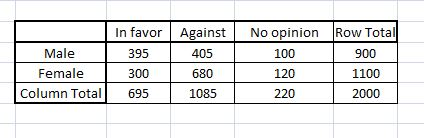# A group of 2,000 randomly selected adults were asked if they are in favor of or against cloning....

## Question:

A group of 2,000 randomly selected adults were asked if they are in favor of or against cloning. The following table gives the responses.

In Favor Against No Opinion
Male 395 405 100
Female 300 680 120

a) If one person is selected at random from these 2,000 adults, find the probability that this person is:

i. in favor of cloning

ii. against cloning

iii. in favor of cloning given the person is a female

iv. a male given the person has no opinion

b) Are the events "male" and "in favor" mutually exclusive? What about the events "in favor" and "against"? Why or why not?

c) Are the events "female" and "no opinion" independent? Why or why not?

## Marginal Probability, Independent and Mutually exclusive events.

The Marginal probability is the probability of a single event. If a contingency table is provided, the marginal probability is equal to the total sum across row/column divided by the sample size.

The conditional probability of A given B is given by:

{eq}P\left( {A/B} \right) = \dfrac{{P\left( {A \cap B} \right)}}{{P\left( B \right)}} {/eq}

A and B are said to be independent events if and only if:

{eq}P\left( {A \cap B} \right) = P\left( A \right) \cdot P\left( B \right) {/eq}

Events A and B are said to be mutually exclusive if:

{eq}\begin{align*} A \cap B = \phi \\ P\left( {A \cap B} \right) = 0 \end{align*} {/eq}

## Answer and Explanation:

Given Information:

A group of 2,000 randomly selected adults if they are in favor, against or do not have any opinion about cloning is given in contingency table as follows:

N = 2000.Let M and W denotes the total number of males and females and F denotes a person is in favor of cloning, A denotes a person is against cloning, O denotes the total of persons who have no opinion,(M/O) denotes a male given the person has no opinion ,{eq}P\left( F \right) {/eq}denotes the probability of a person in favor of cloning,{eq}P\left( A \right) {/eq} denotes the probability of person who is against cloning, N(F/M) denotes the number of males who are in favor of cloning ,N(F/W) denotes the number of females who are in favor of cloning ,N(A/M) and N(A/W) denote the number of males and females who are against cloning.

M = 900

W = 1100

O = 220

{eq}\begin{align*} N\left( {W/O} \right) &= 120\\ N\left( {M/O} \right) &= 100 \end{align*} {/eq}

{eq}\begin{align*} N\left( {F/M} \right) &= 395\\ N\left( {F/W} \right) &= 300\\ N\left( {A/M} \right) &= 405\\ N\left( {A/W} \right) &= 680 \end{align*} {/eq}

a)

i. The Probability that a person selected at random is in favor of cloning is given by

{eq}\begin{align*} P\left( F \right) &= \dfrac{{N\left( {F/M} \right) + N\left( {F/W} \right)}}{N}\\ &= \dfrac{{395 + 300}}{{2000}}\\ &= \dfrac{{695}}{{2000}}\\ &= 0.3475 \end{align*} {/eq}

The Probability that a person selected at random is in favor of cloning is 0.3475.

ii. The Probability that a person selected at random is against cloning is given by:

{eq}\begin{align*} P\left( A \right) &= \dfrac{{N\left( {A/M} \right) + N\left( {A/W} \right)}}{N}\\ &= \dfrac{{405 + 680}}{{2000}}\\ &= \dfrac{{1085}}{{2000}}\\ &= 0.5425 \end{align*} {/eq}

The Probability that a person selected at random is against cloning is 0.5425.

iii. The Probability that a person selected at random is in favor of cloning given the person is a female is given by:

{eq}\begin{align*} P\left( {F/W} \right) &= \dfrac{{N\left( {F/W} \right)}}{W}\\ &= \dfrac{{300}}{{1100}}\\ &= 0.272727 \approx 0.2728 \end{align*} {/eq}

The Probability that a person selected at random is in favor of cloning given the person is a female is 0.2728.

iv. The Probability that a person selected at random is a male given the person has no opinion is given by:

{eq}\begin{align*} P\left( {M/O} \right) &= \dfrac{{N\left( {M/O} \right)}}{O}\\ &= \dfrac{{100}}{{220}}\\ &= 0.454545 \approx 0.4546 \end{align*} {/eq}

The Probability that a person selected at random is a male given the person has no opinion is 0.4546.

b. The number of males who are in favor of cloning is 395 it should have no elements in common for two events to be mutually exclusive.

The events in favor and against are mutually exclusive as they have no elements in common between them.

c. The events females and no opinion are independent if

{eq}P(F \cap O) = P\left( F \right) \cdot P\left( O \right) {/eq}

Considering LHS:

{eq}\begin{align*} P(F \cap O) &= \dfrac{{N\left( {F/O} \right)}}{N}\\ &= \dfrac{{120}}{{2000}}\\ &= 0.06 \end{align*} {/eq}

Considering RHS:

{eq}\begin{align*} P\left( W \right) &= \dfrac{{1100}}{{2000}}\\ P\left( O \right) &= \dfrac{{200}}{{2000}}\\ P\left( W \right) \cdot P\left( O \right) &= \dfrac{{1100}}{{2000}} \times \dfrac{{200}}{{2000}}\\ &= 0.0605 \end{align*} {/eq}

{eq}P(F \cap O) \ne P\left( F \right) \cdot P\left( O \right) {/eq}

Since the RHS and LHS are not exactly equal. The events females and no opinion are not independent.

#### Learn more about this topic:Contingency Table: Statistics, Probability & Examples

from High School Algebra II: Tutoring Solution

Chapter 16 / Lesson 11
63K